## VBA Round

The VBA Round Function rounds numbers to a specified number of digits.

## Syntax of the VBA Round Function

The syntax of the VBA Round Function is:

Round(Expression, [Decimal_places]) where:

• Expression – The number to round.
• Decimal_places (Optional) – An integer that specifies the number of decimal places to round. The value must be greater than or equal to 0 (>=0). If blank, the default of 0 is used, which means the function rounds to the nearest integer.

So, let’s look at an example so that you can see how the VBA Round function works, rounding to 1 decimal place:

The result delivered: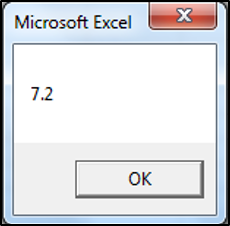## VBA Round a Variable

In the above example, we entered the to-be-rounded number directly into the function, usually however, you’d round a variable instead. The following is an example using a variable instead:

Note: Double is the variable type.

The result delivered: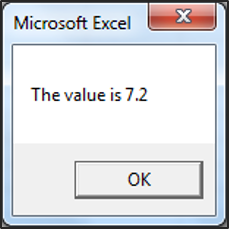### VBA Rounding Results

Actual Number Number of Decimal Places Result
7.25 0 7
7.25 1 7.2
7.25 2 7.25
-7.25 1 -7.2
-7.25 2 -7.25

## VBA Round Cell Value

You can also round a cell value directly in VBA:

## VBA RoundUp Function

Let’s say you want to round a number up, using VBA. There is no built-in VBA RoundUp equivalent function, instead what you can do is call the Excel RoundUp Worksheet function from your VBA code:

Excel’s worksheet functions are available to use in VBA, through the use of the WorksheetFunction object. The only worksheet functions that you can’t call, are those that already have a built-in VBA equivalent.

A reminder of the syntax of the Excel Worksheet RoundUp Function:

ROUNDUP(Number, Digits) where:

• Number – The number that you would like rounded up.
• Digits – The number of digits that you would like to round the number.

So, let’s look at an example, so that you can see how to access the RoundUp Worksheet function in your VBA code:

The result delivered: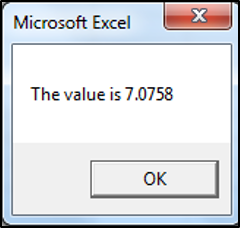### RoundUp to the Nearest Whole Number

You can round up to the nearest whole number by specifying 0 as the number of decimal places:

The result delivered: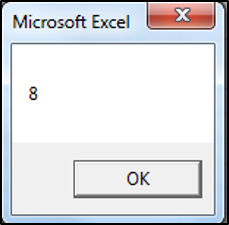### RoundUp Function Results

Actual Number Digits Result
7.075711 0 8
7.075711 1 7.1
7.075711 2 7.08
7.075711 3 7.076
7.075711 -1 10
7.075711 -2 100
7.075711 -3 1000

## VBA RoundDown Function

Let’s say you want to round a number down, using VBA. There is no built-in VBA RoundDown equivalent function either, instead again, what you would do is call the Excel RoundDown Worksheet function from your VBA code.

A reminder of the syntax of the Excel Worksheet RoundDown Function:

ROUNDDOWN(Number, Digits) where:

• Number – The number that you would like rounded down.
• Digits – The number of digits that you would like to round the number.

So, let’s look at an example, so that you can see how to access the RoundDown Worksheet function in your VBA code:

The result delivered: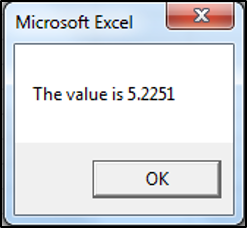### RoundDown to the Nearest Whole Number

You can round down to the nearest whole number by specifying 0 as the number of decimal places:

The result delivered is: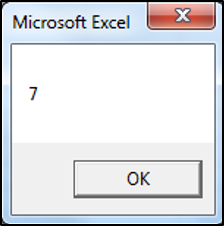### RoundDown Function Results

Actual Number Digits Result
5.225193 0 5
5.225193 1 5.2
5.225193 2 5.22
5.225193 3 5.225
5.225193 -1 0
5.225193 -2 0
5.225193 -3 0

## Other VBA Rounding Functions

### VBA Ceiling – RoundUp to A Specified Significance

VBA does not have a Ceiling.Math function equivalent, so if you want to round a number up to the nearest integer or to the nearest specified multiple of significance, then you can call the Ceiling.Math worksheet function from your VBA code.

A reminder of the syntax of the Excel Worksheet Ceiling.Math Function:

CEILING.MATH(Number, [Significance], [Mode]) where:

• Number – The number that you would like to round up.
• Significance (Optional) – The multiple to which you want your number to be rounded to.
• Mode (Optional) – Controls whether negative numbers are rounded towards or away from zero.

So, let’s look at an example, so that you can see how to access the Ceiling.Math Worksheet function in your VBA code:

The result delivered: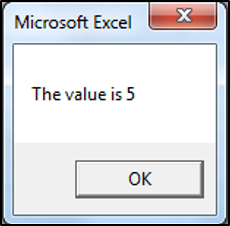### VBA RoundUp To Specified Significance Results

Actual Number Significance Mode Result
4.1221 5
4.1221 3 6
4.1221 50 50
-4.1221 3 -3
-4.1221 3 -1 -6

### VBA Floor – RoundDown to A Specified Significance

VBA does not have a Floor.Math function equivalent either. However, once again, if you want to round a number down to the nearest integer or to the nearest specified multiple of significance, then you can call the Floor.Math worksheet function from VBA.

A reminder of the syntax of the Excel Worksheet Floor.Math Function:

FLOOR.MATH(Number, [Significance], [Mode]) where:
• Number – The number that you would like to round down.
• Significance (Optional) – The multiple to which you want your number to be rounded to.
• Mode (Optional) – Controls whether negative numbers are rounded towards or away from zero.

So, let’s look at an example, so that you can see how to access the Floor.Math Worksheet function in your VBA code:

The result delivered: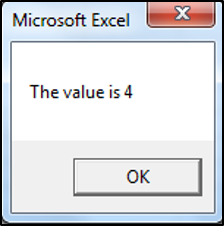### VBA RoundDown to Specified Significance Results

Actual Number Significance Mode Result
4.55555559 4
4.55555559 3 3
4.55555559 50 0
-4.55555559 3 -6
-4.55555559 3 -1 -3

#### You may also like some of this related content...

VBA has many string functions that will allow you to manipulate and work with text
While working in VBA, you often need to convert strings into lowercase, uppercase or proper
Every character in Excel has an assigned number code to itself. The Chr function in
In this article, you will learn how to use the IsEmpty function in VBA to
The following UDF will return the formulae for a given cell reference: VBA Function to